/

### The non-central chi-squared distribution

Distribution name

Non-central chi-squared distribution

Common notation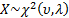Parameters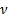= degrees of freedom (positive integer)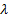= non-centrality parameter (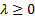)

Domain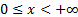Probability density function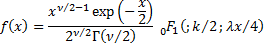Cumulative distribution function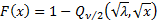where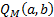is the Marcum-Q function.

Mean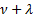Variance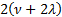Skewness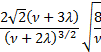(Excess) kurtosis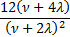Characteristic function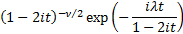The non-central chi-squared distribution withdegrees of freedom and non-centrality parameteris the distribution of the sum of the squares ofindependent normal distributions each with unit standard deviation but with non-zero means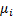where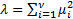. The (standard) chi-squared distribution is a special case of it with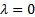.

Nematrian web functions

This distribution is not currently supported within the Nematrian web function library. For details of other supported probability distributions see here.